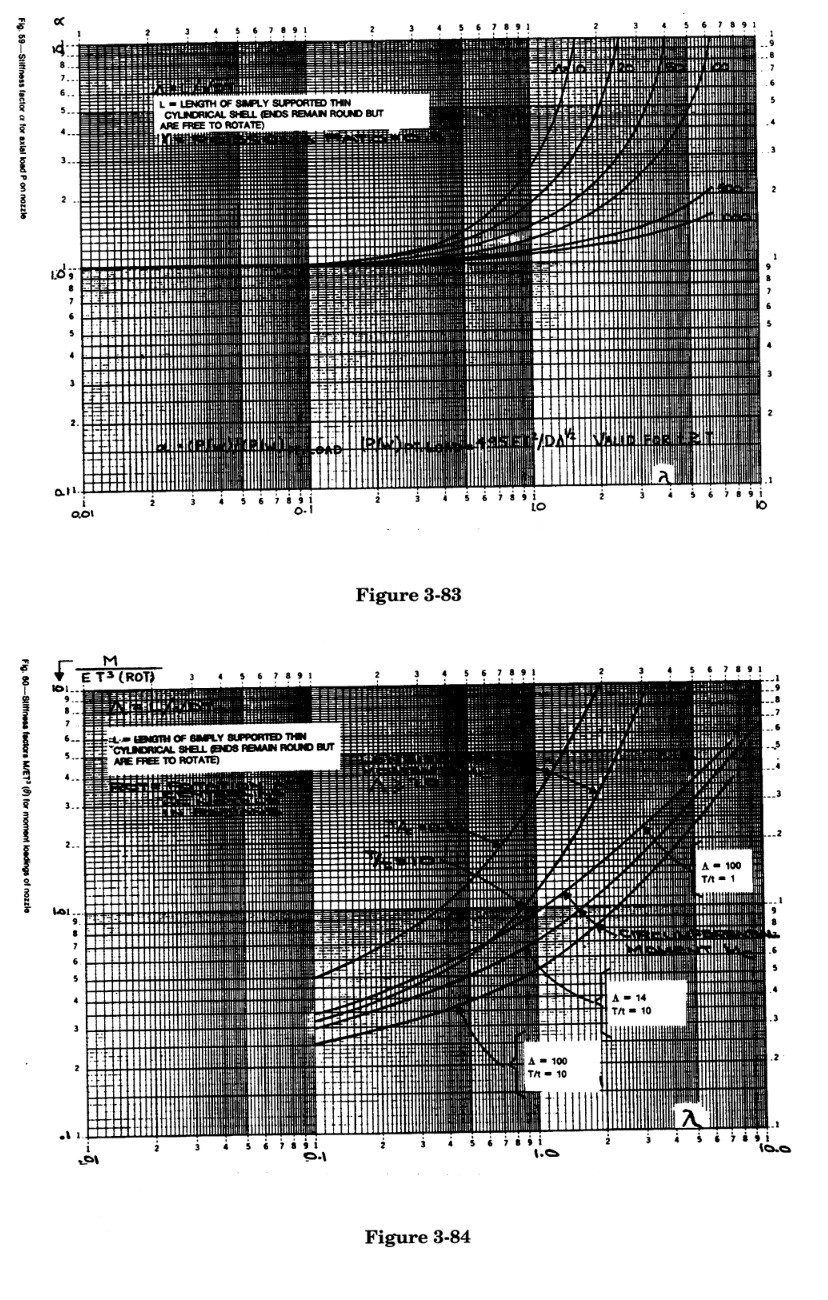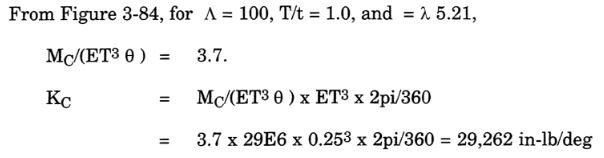Search

# 3.4.3.1 Use of WRC Bulletin 297 in CAESAR II

WRC 297 indicates that a typical nozzle flexibility is equivalent to the flexibility of 3 to 13 elbows concentrated as a point spring at the junction of the pipe with the vessel. It is the opinion of the developers that the results of the WRC 297 flexibility calculations are within one order of magnitude of the exact flexibility solution, where the exact solution is defined by a plate finite element analysis of the model geometry.

The bulletin provides for calculation of flexibilities in the three directions shown in Figure 3-82. All other directions (i.e., torsional, longitudinal shear, and circumferential shear) are considered to be rigid.WRC 297 limits configurations to the following conditions:

d/D < 0.5

d/t > 20

20 < D/T < 2500

d/T > 5

Where:

d = outside diameter of nozzle, in

D = mean diameter of vessel, in

t = thickness of nozzle, in

T = thickness of vessel, in

The particular checks on the nozzle diameter and thickness are not that critical when dealing with flexibilities (i.e., solid plug junctions are not that much more or less flexible than hollow nozzle junctions). The effect of reinforcing pads should be considered if the width of the pad is at least 1.65(DT/2)^1/2. In that case, the vessel wall should be increased to Twall + Tpad.

The WRC 297 nozzle stiffnesses come from three values extracted from the two curves shown in Figures 3-83 and 3-84. These values (α, ML/ (ET^3 θ), and MC/ (ET^3 θ)) are based upon the following geometric parameters:The first step is to check the WRC 297 limitations in order to determine whether it can apply to this situation:

d/D = 18 / 47.75 = 0.377 < 0.5

d/t = 18/0.25 = 72 >20

D/T = 47.75 / 0.25 = 191 < 2500

d/T = 18/0.25 = 72 > 5

Therefore this nozzle/vessel configuration meets the requirements of the WRC 297 bulletin. The stiffness calculations are:From Figure 3-84, the curves are off of the scale for the longitudinal moment, so KL can be assumed to be rigid.CAESAR II provides a feature, accessed from the Kaux menu of the input spreadsheet, whereby the user may define nozzle and vessel parameters for a WRC 297 flexibility calculation. If a nozzle has been defined in the input, during error checking CAESAR II automatically performs the WRC 297 calculations, including lookup of the stiffness parameters from the digitized curves. A zero-length element with the calculated stiffnesses is then automatically inserted into the piping model at the nozzle location between the pipe and the vessel. The CAESAR II input and stiffness calculations (produced during input error checking) for the Figure 3-85 example are shown in Figures 3-86 and 3-87 respectively.